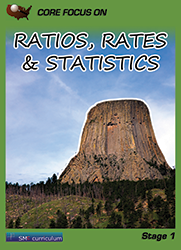#### Ratios, Rates & Statistics

In this course, students learn about ratio concepts and use ratio and rate reasoning to solve problems.  Students develop an understanding of statistical variability.  They also learn to summarize and describe data distributions.

### Textbook & Stage I Series Pricing

1 FREE Teacher Edition(TE) and Teacher Resource Binder(TR) per 40 student texts ordered!